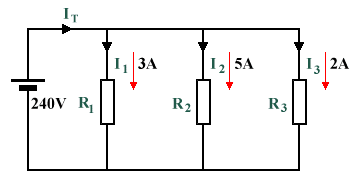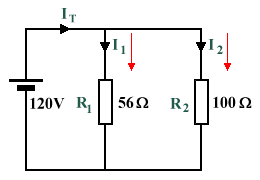# The Current Divider

In the left-hand figure in the diagram below, three resistors are connected in parallel with a battery, E. Unlike a circuit in which the resistors are connected in series, the total resistance of the circuit cannot be obtained simply by adding the three resistances together. In the right-hand figure, a single resistor with a value of RTOTAL is used to provide the same load resistance as the three resistors on the left-hand side, and its resistance value will be smaller than that of the smallest of the three resistors in the original circuit. The total current flowing through the two circuits is exactly the same, but in the left-hand version the current will be split between the three branches. The amount of current flowing through each branch will be inversely proportional to the resistance value of its resistor in relation to the total circuit resistance. Note also that the voltage drop across each resistor will be the same, and will be equal in magnitude to the supply voltage provided by E.These circuits are equivalent in terms of total resistance and current

The sum of the currents flowing through each branch of a parallel circuit is equal to the total current flow in the circuit, and in accordance with Ohm's law will be equal to the supply voltage divided by the total resistance. It is often useful to be able to divide the current in a circuit into multiple branches in order to supply a range of circuit components with the correct amount of current for each component. The relationship between the total circuit current and the current in any one branch of a parallel circuit can be formalised as follows:

 IX  = RT ×  IT RX

In the above equation, IX is the current flowing through the branch being considered, RX is the value of the resistor in that branch, RT is the total circuit resistance, and IT is the total current flowing through the circuit. The relationship between the total resistance and the resistance through each branch of the circuit can be defined using the following formula:

 1 = 1 + 1 + 1 · · · RT R1 R2 R3

To ensure that the correct current flows through each branch of a parallel circuit, a resistor of the correct value must be used in each branch. Note that in real-world circuits, it is not always possible to provide the exact resistance required using a standard resistor value. The options are to use a single standard resistor of approximately the required value, or to use two or more standard resistors in series with a combined resistance that exactly matches the required value. Bear in mind that if other circuit components are introduced into a branch, these will also have some resistance, albeit often small. But how would we work out the value of the resistance needed in each branch in order to provide the correct current? Consider the circuit shown below. The circuit has three resistors in parallel, and each branch is drawing a different current. The supply voltage in this case is 240 V.A typical potential divider consisting of two resistors in series

Total current IT  =  3 A  +  5 A  +  2 A  =  10 A (Kirchhoff's Current Law)

Total resistance  =  240 V / IT  =  240/10  =  24 Ω (Ohm's Law)

R1  =  240 V / 3 A  =  80 Ω (Ohm's Law)

R2  =  240 V / 5 A  =  48 Ω (Ohm's Law)

R3  =  240 V / 2 A  =  120 Ω (Ohm's Law)

Substituting these resistance values into the above formula should confirm that the values obtained are correct.

## Problems

1. In the circuit illustrated below, two resistors are in parallel with a 120 V supply voltage. If the resistance values of R1 and R2 are 56 Ω and 100 Ω respectively, find the current flowing through each resistor (I1 and I2), the total current flowing through the circuit (IT), and the total circuit resistance (RT).A potential divider consisting of two resistors in parallel with a supply voltage

2. In the circuit illustrated below, three resistors are in parallel with a 24 V supply voltage. If the resistance values of R1, R2 and R3 are 15 Ω, 20 Ω and 10 Ω respectively, find the current flowing through each resistor (I1, I2 and I3), the total current flowing through the circuit (IT), and the total circuit resistance (RT).A potential divider consisting of three resistors in parallel with a supply voltage